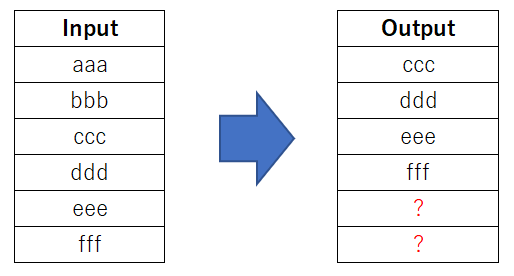# Add rows at the end of the table

Hi Guys,

Having a hard time with adding rows at the end of the table. Currently, I am using RowOutput from node.streamable. Here is my code:

``````public void runFinal(final PortInput[] inputs, final PortOutput[] outputs,
final ExecutionContext exec) throws Exception {
long counter = 0;
int maxLag = m_configuration.getLagInterval() * m_configuration.getLag();
RingBuffer ringBuffer = new RingBuffer(maxLag);
RowInput input = (RowInput)inputs;
RowOutput output = (RowOutput)outputs;
int skippedFirstCount = !m_configuration.isSkipInitialIncompleteRows() ? -1
: m_configuration.getLagInterval() * m_configuration.getLag();
DataRow row;
while ((row = input.poll()) != null) {
if (counter >= skippedFirstCount) {
output.push(copyWithNewCells(row, newCells));
``````

I used Lag column node’s code as my reference. Based on my understanding, its the output.push() that is doing the adding of rows. Or if I am looking at the wrong codes in adding new rows, please do enlighten me. Please image below for the output data that I want to achieve.In this scenario, if the user sets “2” as the number of rows to add, it will add two rows then it will adjust upward.

Br,
Gambit

Hi @Gambit,

Yes `output.push()` is the method you need to call to add additional rows to your output table.
You should be able to just call this with missing nodes after finishing the main part of execution.
Take a look how it is done in the Lag node:
https://bitbucket.org/KNIME/knime-base/src/10be75725833da035f3004ffb008e4ccdba8b9fe/org.knime.base/src/org/knime/base/node/preproc/columnlag/LagColumnStreamableOperator.java?at=master#lines-162:171

best,
Gabriel

1 Like

Hi @gab1one ,

Thanks! Is there a method to add the new rows at the end of the table like the image I showed? Because I can only add the rows at the beginning of the table.

Thanks!

Br,
Gambit

Hi @Gambit

The lines I linked, add lines to the end of the table. Whenever you call `push(..)` on a `RowOutput` the given row is added to the end of the created table.

best,
Gabriel

1 Like

Hi @gab1one ,

I see. However, I can’t find the part of the code that tells that the rows where it should be added. It always start at the first row or row 0, in case I need it to add the new rows at the last row of the table, how should I do it? Let’s say my last Row is row 5.

Thank you for the support!

Br,
Gambit

You can’t specify where to add a row directly. In a `RowOutput` you always add to the end of the table being created.
If you want to move e.g. the first 5 rows of a table to it’s end, you will need to store them in e.g. a list, then push all the other rows directly to the output, and finally add the rows you stored to the end.
Usually you create a new output table by appending rows to either a RowOutput or a DataContainer, these datastructures do not allow you to add rows anywhere but the end of the table.

Hi @gab1one ,

You mean RowOutput can do either of these two outputs?

It was like shifting or adjusting the rows, either downward or upward. The new rows are always added either top or bottom ends of the column but not anywhere at the middle. Or if RowOutput can only add rows at the top end of the column, do you have any suggestions? My first idea is to reverse the rows before adding the new rows with null values then I will reverse it back again so the new rows will be placed at the bottom end of the column.

Br,
Gambit

Hi @Gambit,
I think there is a little misunderstanding going on here about the capabilities of the RowOutput.
When using a RowOutput, it is totally up to you with which rows to fill it, the only constraint that you have, is that you can only append to it.

For your usecase I’d suggest something like the following pseudo code:

``````// push empty rows
for (int i = 0; i < 2; i++){
Row emptyRow = makeEmptyRow();
rowOutput.push(emptyRow);
}

// copy input rows
DataRow row;
while ((row = input.poll()) != null) {
output.push(row);
}
output.close();
``````

But you don’t need to just copy the input. If you e.g. want to change every other row, you could do that easily by adjusting the loop:

``````
// copy input rows
DataRow row;
int counter = 0;
while ((row = input.poll()) != null) {
if(counter % 2 == 0) {
output.push(row);
} else {
output.push(modifyRow(row)); // call to your custom modification function
}
counter++;
}
``````

I hope this gives you an idea on how you can get creative with the iteration.

1 Like

Hi @gab1one ,

Thank you for this. Regarding the target index, based on the lag column node, is this the codes that decides what index or row to add the new null values and adjust the existing rows?

private static final class RingBuffer {

``````    private final int m_capacity;
private final ArrayList<DataCell> m_storageList;
int m_nextIndex = 0;

RingBuffer(final int capacity) {
m_storageList = new ArrayList<DataCell>(capacity);

m_capacity = capacity;
for (int i = 0; i < capacity; i++) {

}
}

System.out.println(cell);
Collections.reverse(Arrays.asList(cell));
m_storageList.set(m_nextIndex, cell);
m_nextIndex += 1;
if (m_nextIndex >= m_capacity) {
m_nextIndex = 0;
}

}

DataCell get(final int requestIndex) {
int index = m_nextIndex - requestIndex;
if (index < 0) {
index += m_capacity;
}
return m_storageList.get(index);

}

}
``````

}